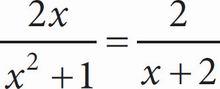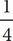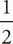# SAT Math Multiple Choice Question 16: Answer and Explanation

### Test Information

Question: 16

2. If, what is the value of x ?

• A. -• B.• C. 0
• D. 2

B To solve this equation, use cross multiplication to get (2x)(x + 2) = (x2 + 1)(2). Expand the equation to get 2x2 + 4x = 2x2 + 2. Once you combine like terms, the result is 2x2 - 2x2 + 4x = 2 or 4x = 2. Solve for x by dividing both sides by 4 to get x =, which is (B).